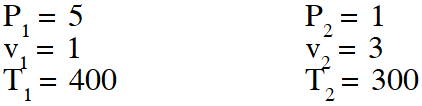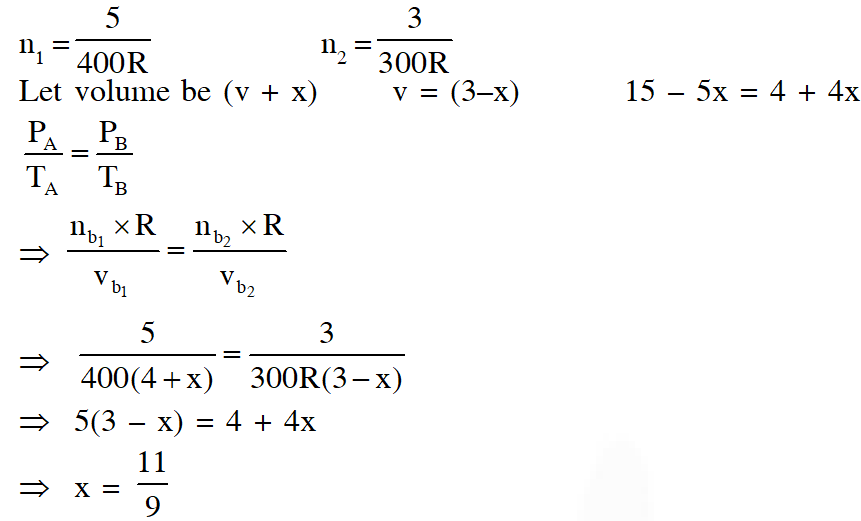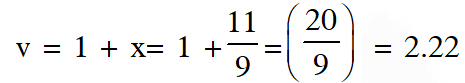Most Affordable JEE | NEET | 8,9,10 Preparation by Kota's Top IITian Doctor Faculties

# States of Matter - JEE Advanced Previous Year Questions with Solutions`
JEE Advanced Previous Year Questions of Chemistry with Solutions are available at eSaral. Practicing JEE Advanced Previous Year Papers Questions of Chemistry will help the JEE aspirants in realizing the question pattern as well as help in analyzing weak & strong areas. Simulator   Previous Years JEE Advance Questions
Q. At 400 K, the root mean square (rms) speed of a gas X (molecular weight = 40) is equal to the most probable speed of gas Y at 60 K. The molecular weight of the gas Y is - [JEE 2009]
Ans. 4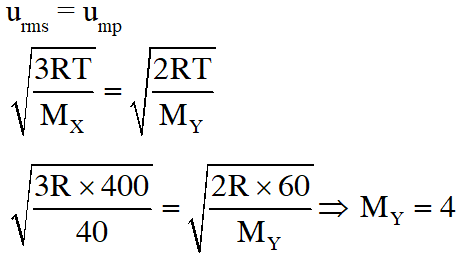Q. The term that corrects for the attractive forces present in a real gas in the Vander Waals' equation is - (A) nb (B) $\frac{\mathrm{an}^{2}}{\mathrm{V}^{2}}$ $(\mathrm{C})-\frac{\mathrm{an}^{2}}{\mathrm{V}^{2}}$ (D) –nb [JEE 2009]
Ans. (B) Attractive force term of real gas is represented by $\frac{\mathrm{n}^{2} \mathrm{a}}{\mathrm{v}^{2}}$
Q. To an evacuated vessel with movable piston under external pressure of 1 atm., 0.1 mol of He and 1.0 mol of an unknown compound (vapour pressure 0.68 atm. at $\left.0^{\circ} \mathrm{C}\right)$) are introduced. Considering the ideal gas behaviour, the total volume (in litre) of the gases at $0^{\circ} \mathrm{C}$ is close to - [JEE 2011]
Ans. 7 PV = nRT 0.32 × V = 0.1 × 0.0821 × 273 V = 7 litre
Q. For one mole of a van der Waals' gas when b = 0 and T = 300 K, the PV vs. 1/V plot is shown below. The value of the van der Waals' constant a (atm. litre $^{2} \mathrm{mol}^{-2}$) is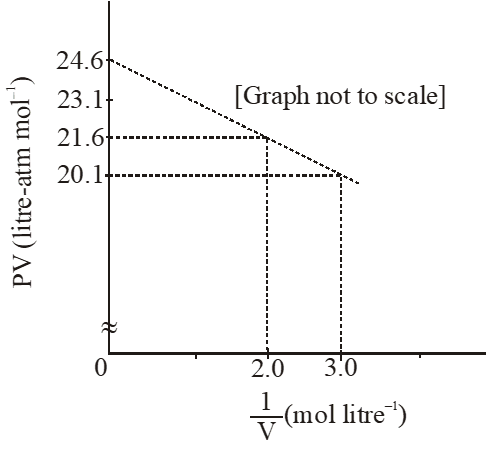(A) 1.0 (B) 4.5 (C) 1.5 (D) 3.0 [JEE 2012]
Ans. (C) If $\mathrm{b}=0 ;\left(\mathrm{P}+\frac{\mathrm{a}}{\mathrm{Vm}^{2}}\right) \times \mathrm{Vm}=\mathrm{RT}$ $\mathrm{PVm}+\frac{\mathrm{a}}{\mathrm{Vm}}=\mathrm{RT}$ $\mathrm{PVm}+\frac{\mathrm{a}}{\mathrm{Vm}}=\mathrm{RT}$ of the form, y = c + mx here, m = –a $\mathrm{m}=\frac{\mathrm{y}_{2}-\mathrm{y}_{1}}{\mathrm{x}_{2}-\mathrm{x}_{1}}=\frac{21.6-20.1}{2-3.0}=-1.5$ $\therefore \mathrm{a}=1.5 \mathrm{atm} \mathrm{L}^{2} \mathrm{mol}^{-2}$
Paragraph for Question 5 & 6 X and Y are two volatile liquids with molar weights of 10g $\operatorname{mol}^{-1}$ and 40g $\operatorname{mol}^{-1}$ respectively. Two cotton plugs, one soaked in X and the other soaked in Y, are simultaneously placed at the ends of a tube of length L = 24 cm, as shown in the figure. The tube is filled with an inert gas at 1 atmosphere pressure and a temperature of 300K. Vapours of X and Y react to form a product which is first observed at a distance d cm from the plug soaked in X. Take X and Y to have equal molecular diameters and assume ideal behaviour for the inert gas and the two vapours.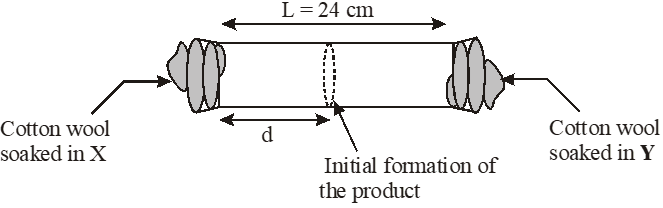Q. The value of d in cm (shown in the figure), as estimated from Graham's law, is – (A) 8 (B) 12 (C) 16 (D) 20 [JEE 2014]
Ans. (C)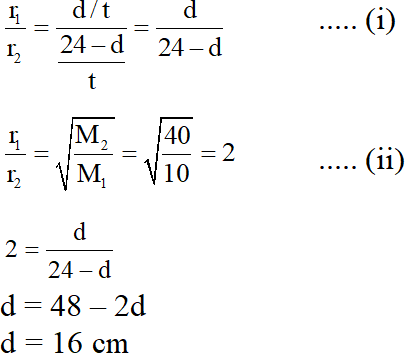Q. The experimetnal value of d is found to be smaller than the estimate obtained using Graham's law. This is due to – (A) Larger mean free path for X as compared to that of Y (B) Larger mean free path for Y as compared to that of X (C) Increased collision frequency of Y with the inert gas as compared to that of X with the inert gas (D) Increased collision frequency of X with the inert gas as compared to that of Y with the inert gas [JEE 2014]
Ans. (D)
Q. One mole of a monoatomic real gas satisfies the equation P(V– b) = RT where b is a constant. The relationship of interatomic potential V(r) and interatomic distance r for the gas is given by – (A)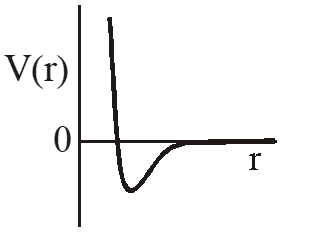(B)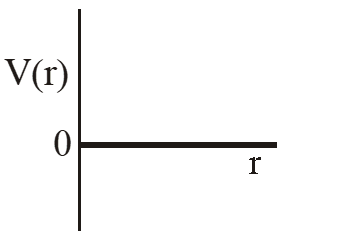(C)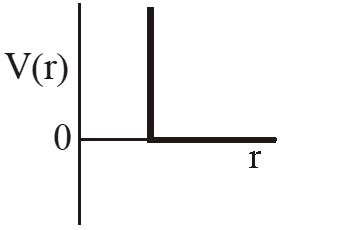(D)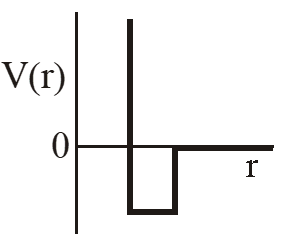[JEE 2015]
Ans. (C) P(v – b) = RT Using thermodynamic equation of state, we get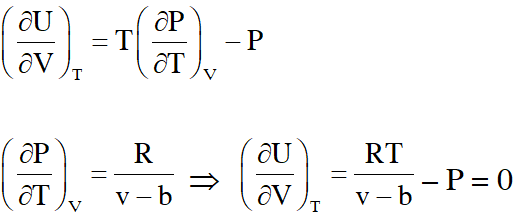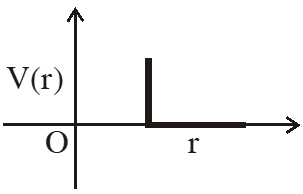Q. The diffusion coefficient of an ideal gas is proportional to its mean free path and mean speed. The absolute temperature of an ideal gas is increased 4 times and its pressure is increased 2 times. As a result, the diffusion coefficient of this gas increases x times. The value of x is [JEE - Adv. 2016]
Ans. 4 Rate of diffusion $\propto \lambda \times U_{\text {Avg }}$ $\propto \frac{1}{\sqrt{2} \pi \sigma^{2} \mathrm{N}^{*}} \times \mathrm{U}_{\mathrm{Avg}}$ $\propto \frac{\mathrm{U}_{\mathrm{Avg}}}{\sqrt{2} \pi \sigma^{2} \mathrm{N}^{*}}$ $\propto \frac{\mathrm{U}_{\mathrm{Avg}}(\mathrm{kT})}{\sqrt{2 \pi \sigma^{2} \mathrm{P}}}$ Rate of diffusion $\propto \frac{\mathrm{T}^{\frac{3}{2}}}{\mathrm{P}}$ $\frac{\mathrm{r}_{\mathrm{final}}}{\mathrm{r}_{\mathrm{initial}}}=\frac{(4)^{\frac{3}{2}}}{2}$ $\frac{\mathrm{r}_{\text {final }}}{\mathrm{r}_{\text {inital }}}=4$
Q. A closed tank has two compartments A and B, both filled with oxygen (assumed to be ideal gas). The partition separating the two compartments is fixed and is a perfect heat insulator (Figure 1). If the old partition is replaced by a new partition which can slide and conduct heat but does NOT allow the gas to leak across (Figure 2), the volume (in $m^{3}$ ) of the compartment A after the system attains equilibrium is____.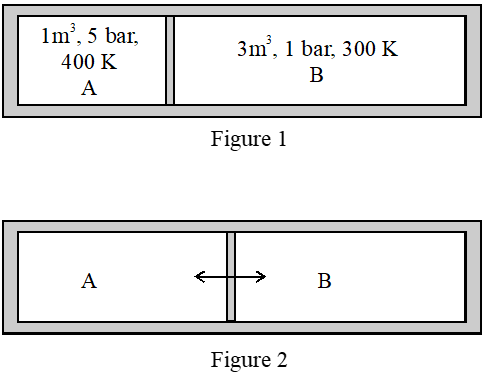[JEE - Adv. 2018]
Ans. -14.6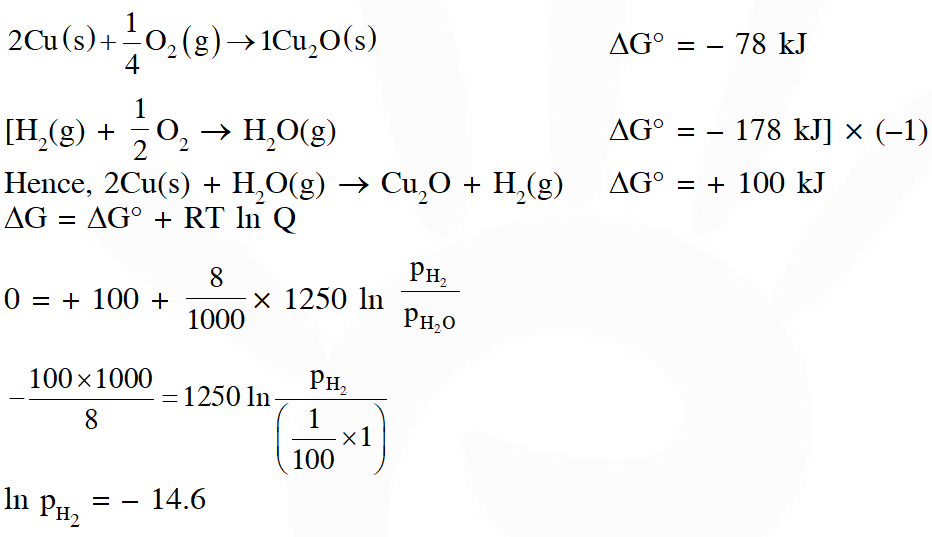Q. The surface of copper gets tarnished by the formation of copper oxide. $\mathrm{N}_{2}$ gas was passed to prevent the oxide formation during heating of copper at 1250 K. However, the N2 gas contains 1 mole % of water vapour as impurity. The water vapour oxidises copper as per the reaction given below : $2 \mathrm{Cu}(\mathrm{s})+\mathrm{H}_{2} \mathrm{O}(\mathrm{g}) \rightarrow \mathrm{Cu}_{2} \mathrm{O}(\mathrm{s})+\mathrm{H}_{2}(\mathrm{g})$ $\mathrm{p}_{\mathrm{H} 2}$ is the minimum partial pressure of $\mathrm{H}_{2}$ (in bar) needed to prevent the oxidation at 1250 K. The value of ln $\left(\mathrm{p}_{\mathrm{H} 2}\right)$ is ___. (Given : total pressure = 1 bar, R (universal gas constant) = 8 $\mathrm{JK}^{-1} \mathrm{mol}^{-1}$ , ln(10) = 2.3. Cu(s) and $\mathrm{Cu}_{2} \mathrm{O}(\mathrm{s})$ are mutually immiscible. At $1250 \mathrm{K}: 2 \mathrm{Cu}(\mathrm{s})+1 / 2 \mathrm{O}_{2}(\mathrm{g}) \rightarrow \mathrm{Cu}_{2} \mathrm{O}(\mathrm{s}) ; \Delta \mathrm{G}^{\theta}=-78,000 \mathrm{J} \mathrm{mol}^{-1}$ $\left.\mathrm{H}_{2}(\mathrm{g})+1 / 2 \mathrm{O}_{2}(\mathrm{g}) \rightarrow \mathrm{H}_{2} \mathrm{O}(\mathrm{g}) ; \Delta \mathrm{G}^{\theta}=-1,78,000 \mathrm{J} \mathrm{mol}^{-1} ; \mathrm{G} \text { is the Gibbs energy }\right)$ [JEE - Adv. 2018]
Ans. 2.22